# INFO Function

Returns information about the current operating environment

## What is the INFO Function?

The INFO function is categorized under Excel Information functions. The function helps extract information about the current operating environment, including the Excel release version and operating system.

Imagine a situation wherein you are working as a remote financial analyst and receive weekly data, in the form of Excel files, from your colleagues based in different countries. As you would be using multiple Excel workbooks, the INFO function helps you extract information about the OS, the number of worksheets in the file, Excel version, etc.

### Formula

=INFO(type_text)

The INFO function uses only one argument:

Type_text (required argument) – This is the text that specifies what type of information you want to be returned.

The following are the seven types of information that can be requested: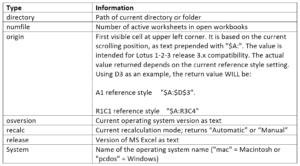### How to use the INFO function in Excel?

As a worksheet function, INFO can be entered as part of a formula in a cell of a worksheet.

To understand the uses of this function, let us consider a few examples:

#### Example 1

Suppose we provide the following INFO formulas :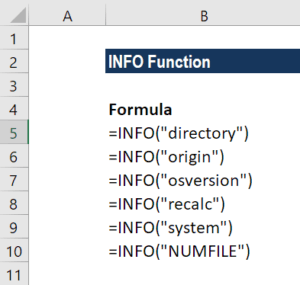Let’s see what this function returns when we use the following examples: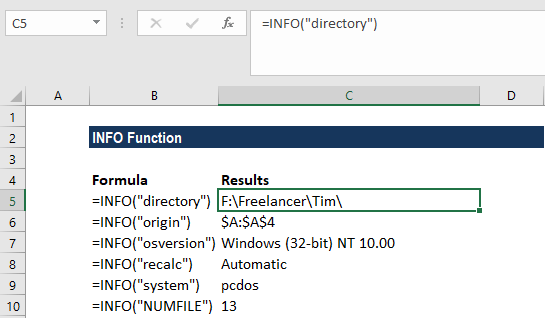“Directory” returned the path of the current folder and “origin” provided information on A1 reference style.

The “osversion” argument provides information on the operating system. “Recalc” helps us understand if calculations are manual or automatic.

“System” provides us with the information that the file was created on Windows.

As the examples were created on Windows, we got the results accordingly. The results may vary depending on the operating system.

#### Example 2

We can add a line break on the basis of the current OS (Mac or Windows). Using the INFO function with CHAR function, we can add a line break. Using the INFO function to test the system, we can ensure the correct break character is used, which is CHAR(10) for Windows and CHAR(13) for MAC.

The formula to be used would be =IF(INFO(“system”) =”pcdos”,CHAR(10),CHAR(13))

We put that in cell F2, as shown below: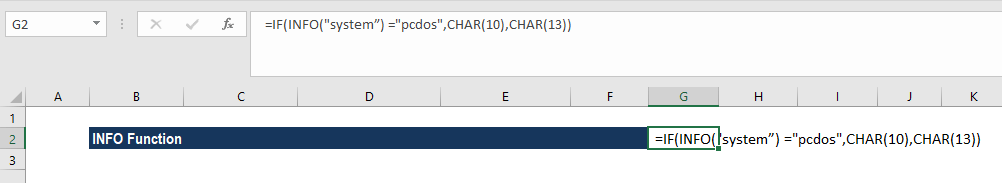We can now add a formula that references F2 so we can use the word break like a variable later. If Excel is running on a Mac, the break will equal CHAR(13). If not, the break will equal CHAR(10).

In column D, we concatenate the address information that appeared in A, B, and C. The formula to be used is shown below: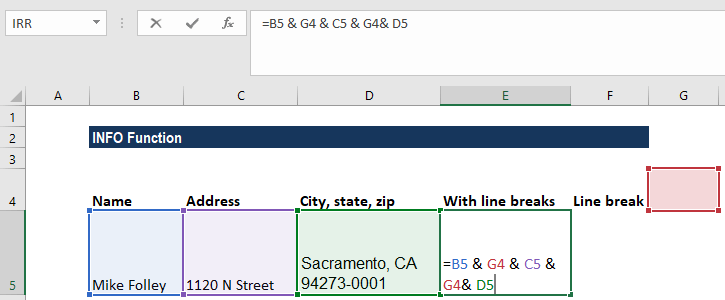The result we get is a concatenation of text with line breaks, as shown below: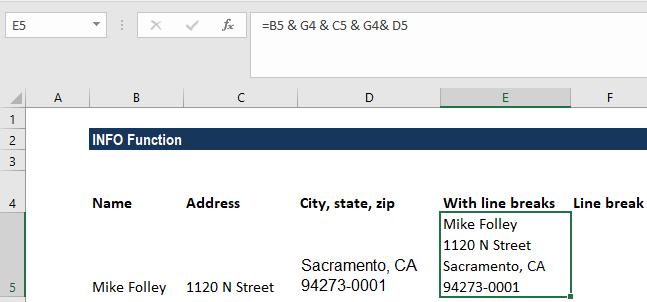### Things to remember about the INFO Function

1. #N/A! – In previous versions of Microsoft Excel, the “memavail,” “memused,” and “totmem” type_text values returned memory information. However, these are no longer supported and now return a #N/A error value.
2. #VALUE! error – Occurs when the type_text argument is any other invalid value.

Thanks for reading CFI’s guide to important Excel functions! By taking the time to learn and master these functions, you’ll significantly speed up your financial analysis. To learn more, check out these additional CFI resources:

• Excel Functions for Finance